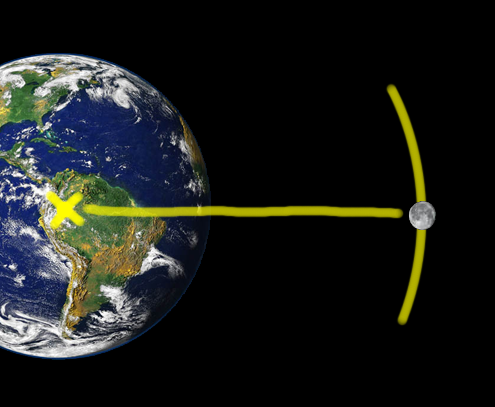DEFCOM1

## Shells and 2d Point Rotation

 Create Shell Variables Edit the update function Edit the draw function

Create Shell Variables

Just like Level 1 Lesson 10 we need to create the variables that will hold the data about the shell

• Position
• Direction
• current status of the shell (not fired or firing)

We do not need to add any more textures as we will be using the same spritesheet as the tank uses.

```protected Vector2 shellPos;
protected float shellRot;
protected int fireState;
```

Edit the update function

We now need to add a fire button, I shall be using the space bar.

But… it should only work if the gun is not already firing.

First we initialise the shell to match the position and direction (rotation of the tank)

We will use a maths equation to rotate the shells starting position around the centre of the tank

Just like the earth rotates around the centre of the earth we will rotate the position of the shell around the centre of the tank (well the base of the barrel, which is where the shell should start from)This is the formula we will be using

shellX = (HorizontalDistance * Cos(angle)) – (VerticalDistance * Sin(angle))

shellY = (HorizontalDistance * Sin(angle)) + (VerticleDistance * Cos(angle))

The following code will check the spacebar and only fire if it is not currently firing.

```if (key.IsKeyDown(Keys.Space) && fireState == 0)
{

//fire
//position shell to barrel
float sx, sy;

//start 70 pixels from centre of the tank (horizontal not vertical)
sx = (70 * (float)Math.Cos(rotation)) - (0 * (float)Math.Sin(rotation));
sy = (70 * (float)Math.Sin(rotation)) + (0 * (float)Math.Cos(rotation));

//set shell position
shellPos.X = position.X + sx;
shellPos.Y = position.Y + sy;

//set rotation of shell to angle of tank
shellRot = rotation;

//FIRE!!!
fireState = 1;

}
```

Then we use this data to move the shell

Moving the shell is the exact same maths as moving the tank

```if (fireState == 1) //IF Firing
{
//move shell position
float x, y;

x = (500 * elapsed) * (float)Math.Cos(shellRot);    //500 is speed of shell
y = (500 * elapsed) * (float)Math.Sin(shellRot);

shellPos.X += x;
shellPos.Y += y;

//if out of screen bounds, change to screen coords!
if (shellPos.X < 0 || shellPos.X > 1280 || shellPos.Y < 0 || shellPos.Y > 720)
{
fireState = 0;//reset shell
}
}
```

When it is out of bounds we should stop/reset the shell

Finally we can draw the shell.

We shall only draw the shell if it is ‘firing’

Put this in to your draw function, just after you draw the tank

```if (fireState == 1)
{
spriteBatch.Draw(SpriteSheet, shellPos, new Rectangle(512, 0, 128, 128), Color.White,
shellRot,new Vector2(64, 64), 1.0f, SpriteEffects.None, 1);

}
```

You should now have a firing tank :)

You could now try adding target to hit!!!

<<Completed Listings>>

```public class Game1 : Microsoft.Xna.Framework.Game
{
GraphicsDeviceManager graphics;
SpriteBatch spriteBatch;

//Global Variables
protected Texture2D SpriteSheet;

protected Vector2 position;
protected float rotation;

protected Vector2 shellPos;
protected float shellRot;
protected int fireState;

public Game1()
{
graphics = new GraphicsDeviceManager(this);
Content.RootDirectory = "Content";
}

protected override void Initialize()
{

position = new Vector2();
rotation = 0;

position.X = 200;
position.Y = 200;

shellPos = new Vector2();
shellRot = 0;
fireState = 0;

base.Initialize();

}

{
// Create a new SpriteBatch, which can be used to draw textures.
spriteBatch = new SpriteBatch(GraphicsDevice);

}

{
// TODO: Unload any non ContentManager content here
}

protected override void Update(GameTime gameTime)
{
// Allows the game to exit
this.Exit();

KeyboardState key = Keyboard.GetState();
float elapsed = (float)gameTime.ElapsedGameTime.TotalSeconds;

if (key.IsKeyDown(Keys.Left))
{
rotation -= 1.0f * elapsed;
}
if (key.IsKeyDown(Keys.Right))
{
rotation += 1.0f * elapsed;
}
if (key.IsKeyDown(Keys.Up))
{
float x, y;

x = (100 * elapsed) * (float)Math.Cos(rotation);
y = (100 * elapsed) * (float)Math.Sin(rotation);

position.X += x;
position.Y += y;
}

if (key.IsKeyDown(Keys.Down))
{
float x, y;

x = (-100 * elapsed) * (float)Math.Cos(rotation);
y = (-100 * elapsed) * (float)Math.Sin(rotation);

position.X += x;
position.Y += y;
}

if (key.IsKeyDown(Keys.Space) && fireState == 0)
{

//fire
//position shell to barrel
float sx, sy;

//start 70 pixels from centre of the tank (horizontal not vertical)
sx = (70 * (float)Math.Cos(rotation)) - (0 * (float)Math.Sin(rotation));
sy = (70 * (float)Math.Sin(rotation)) + (0 * (float)Math.Cos(rotation));

//set shell position
shellPos.X = position.X + sx;
shellPos.Y = position.Y + sy;

//set rotation of shell to angle of tank
shellRot = rotation;

//FIRE!!!
fireState = 1;

}

if (fireState == 1) //IF Firing
{
//move shell position
float x, y;

x = (500 * elapsed) * (float)Math.Cos(shellRot);    //500 is speed of shell
y = (500 * elapsed) * (float)Math.Sin(shellRot);

shellPos.X += x;
shellPos.Y += y;

//if out of screen bounds //change to screen coords
if (shellPos.X < 0 || shellPos.X > 1280 || shellPos.Y < 0 || shellPos.Y > 720)
{
fireState = 0;//reset shell
}
}

base.Update(gameTime);
}

protected override void Draw(GameTime gameTime)
{

GraphicsDevice.Clear(Color.SeaGreen);

spriteBatch.Begin();

spriteBatch.Draw(SpriteSheet, position, new Rectangle(0, 0, 128, 128),
Color.White, rotation, new Vector2(64, 64), 1.0f, SpriteEffects.None, 1);

if (fireState == 1)
{
spriteBatch.Draw(SpriteSheet, shellPos, new Rectangle(512, 0, 128, 128),
Color.White, shellRot, new Vector2(64, 64), 1.0f, SpriteEffects.None, 1);

}

spriteBatch.End();

base.Draw(gameTime);
}
}
```